# RD Sharma Solutions for Class 11 Chapter 23 - The Straight Lines Exercise 23.1

Here, in this exercise, we shall discuss concepts related to slope of a line and angle between two lines. Students who aim to secure good marks in the board exams are advised to refer to RD Sharma Class 11 Maths Solutions. The solutions are designed by our expert faculty team, in a step by step manner, to help students understand the concepts clearly. Students are advised to practice regularly to come out with flying colours in their board examination. The pdf of RD Sharma Solutions are provided in the links given below, which can be downloaded easily and can be used for future reference as well.

## Download the pdf of RD Sharma Solutions for Class 11 Maths Exercise 23.1 Chapter 23 – The Straight Lines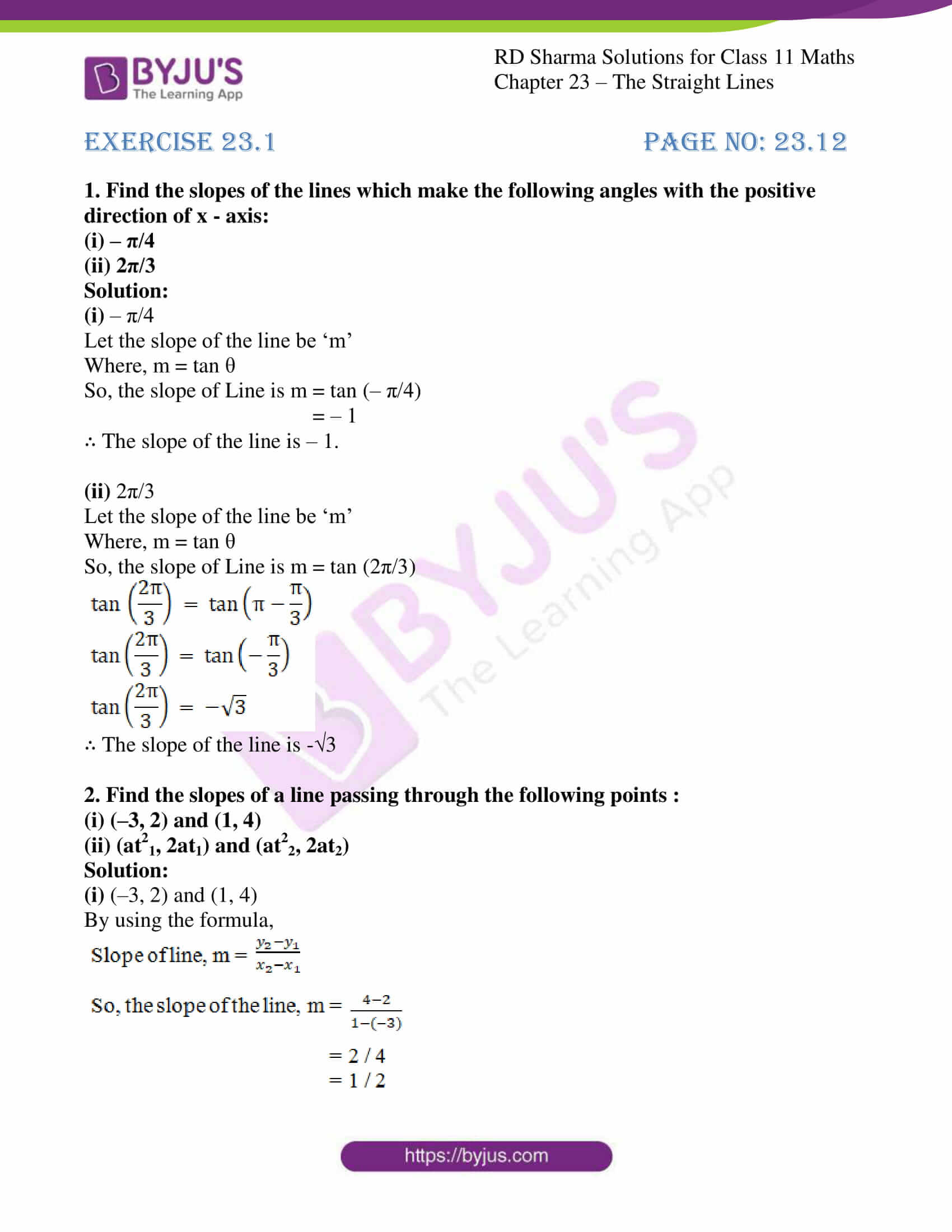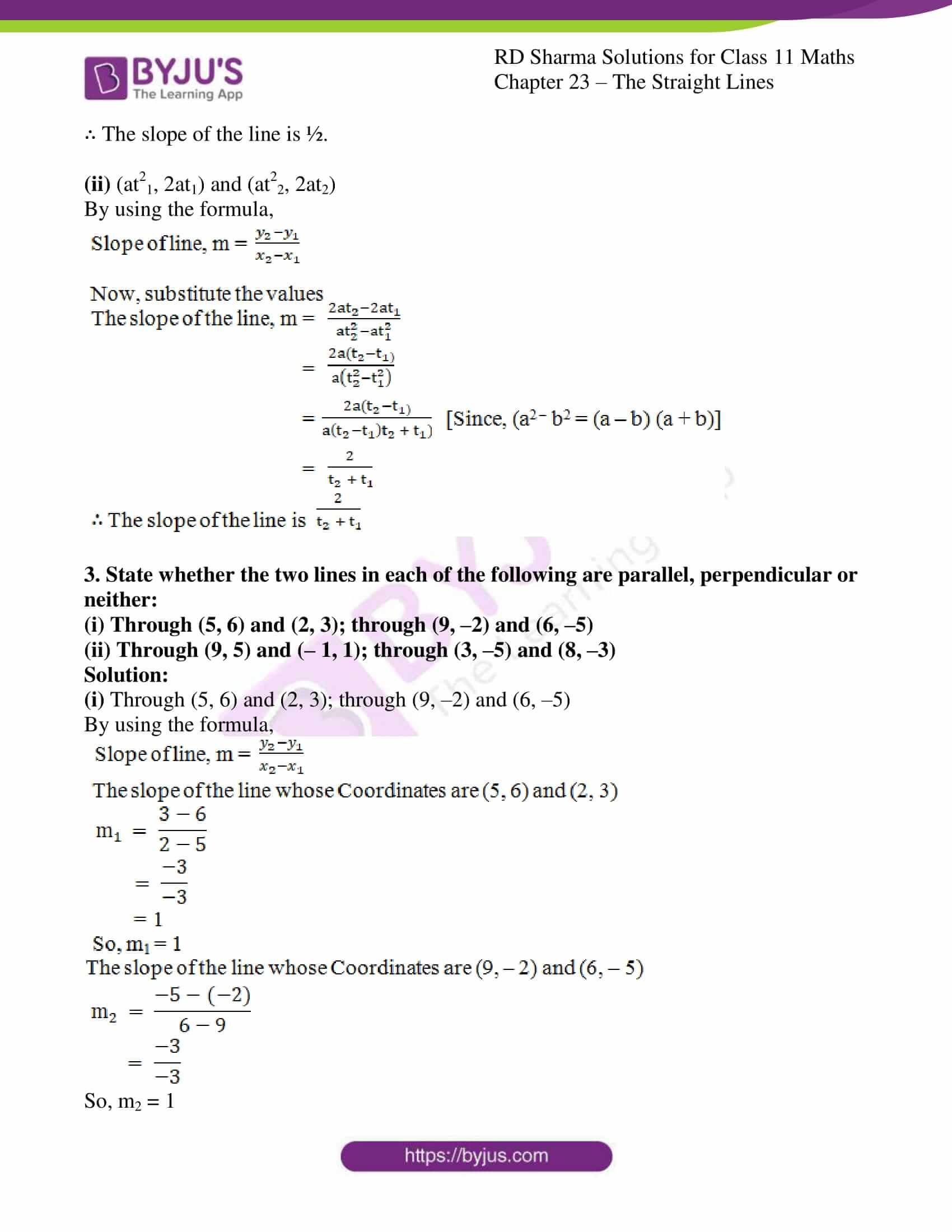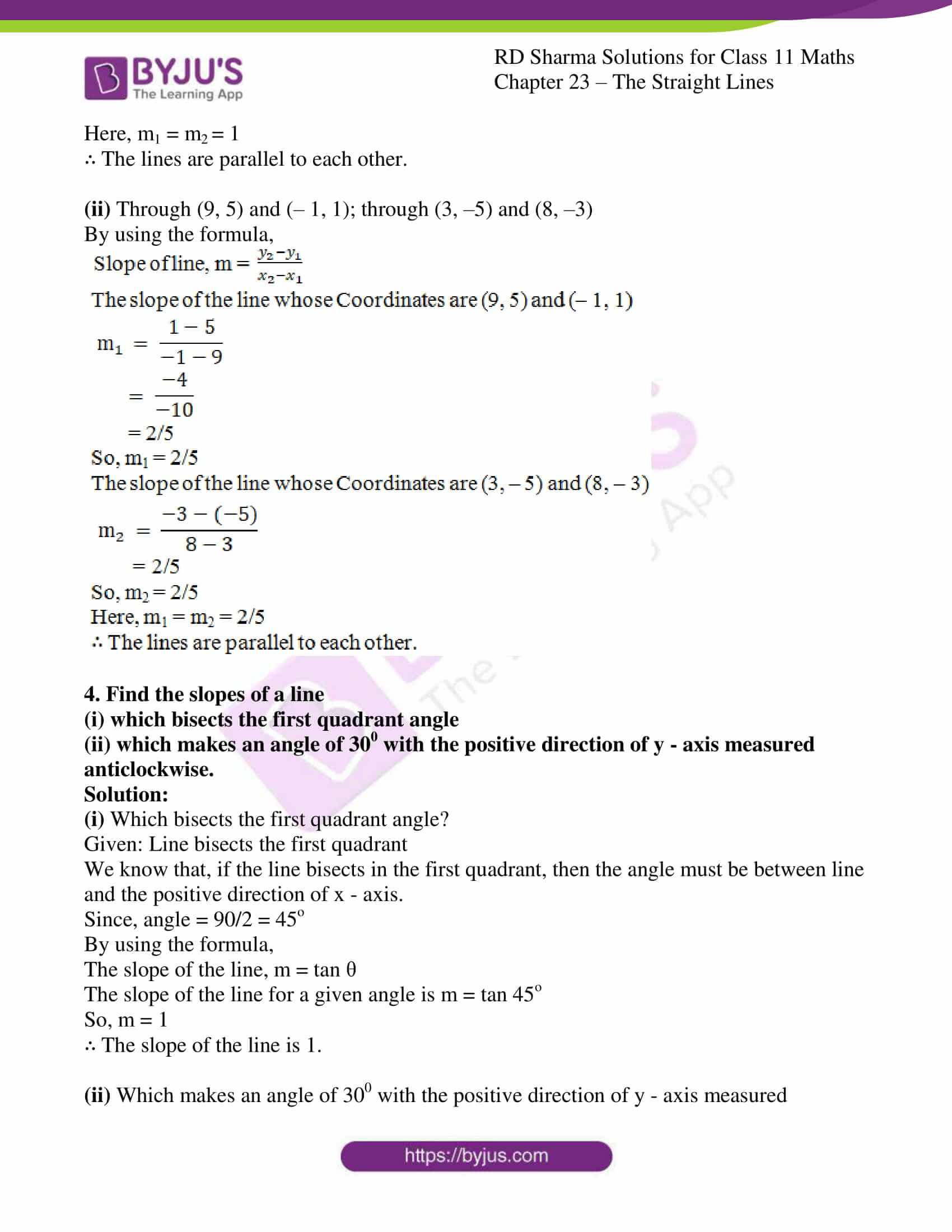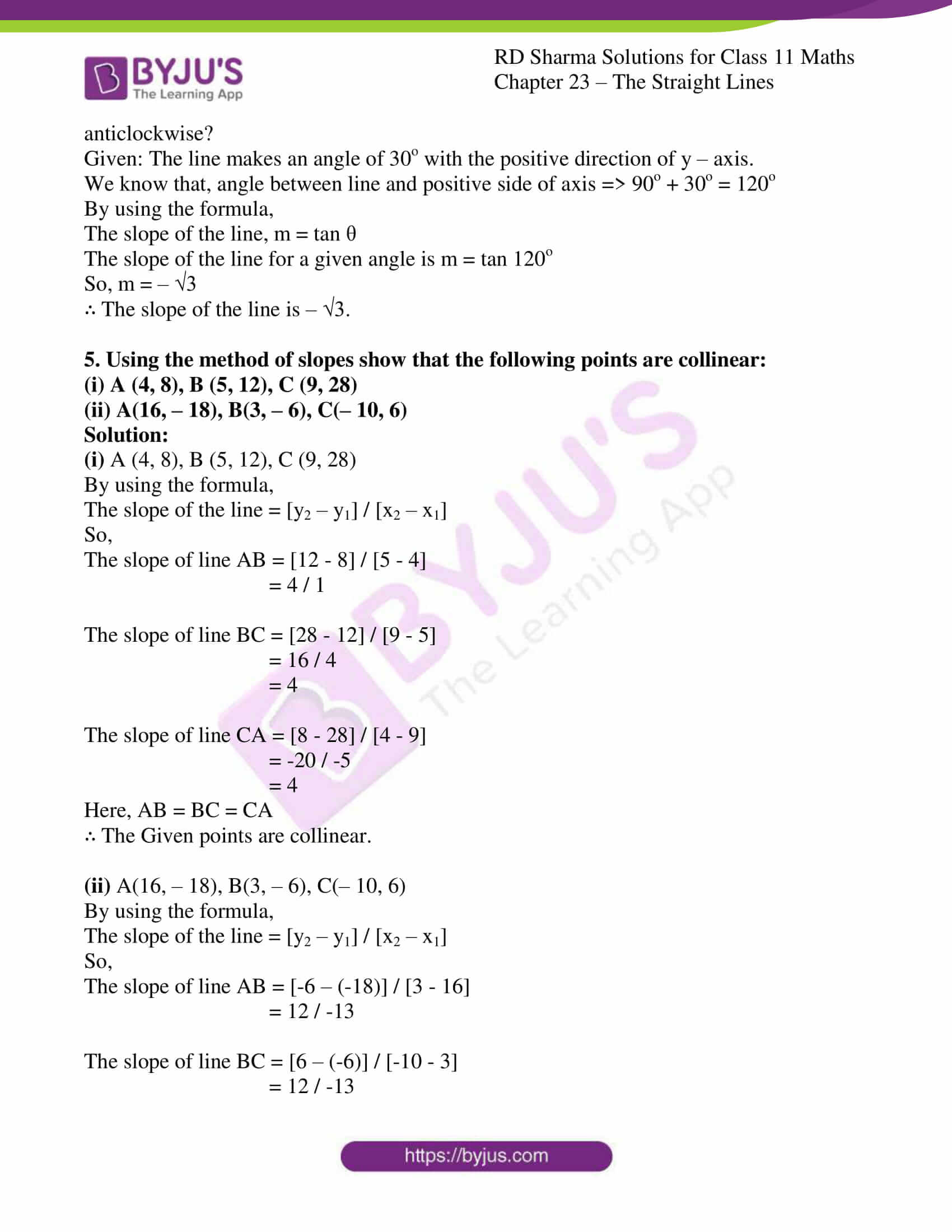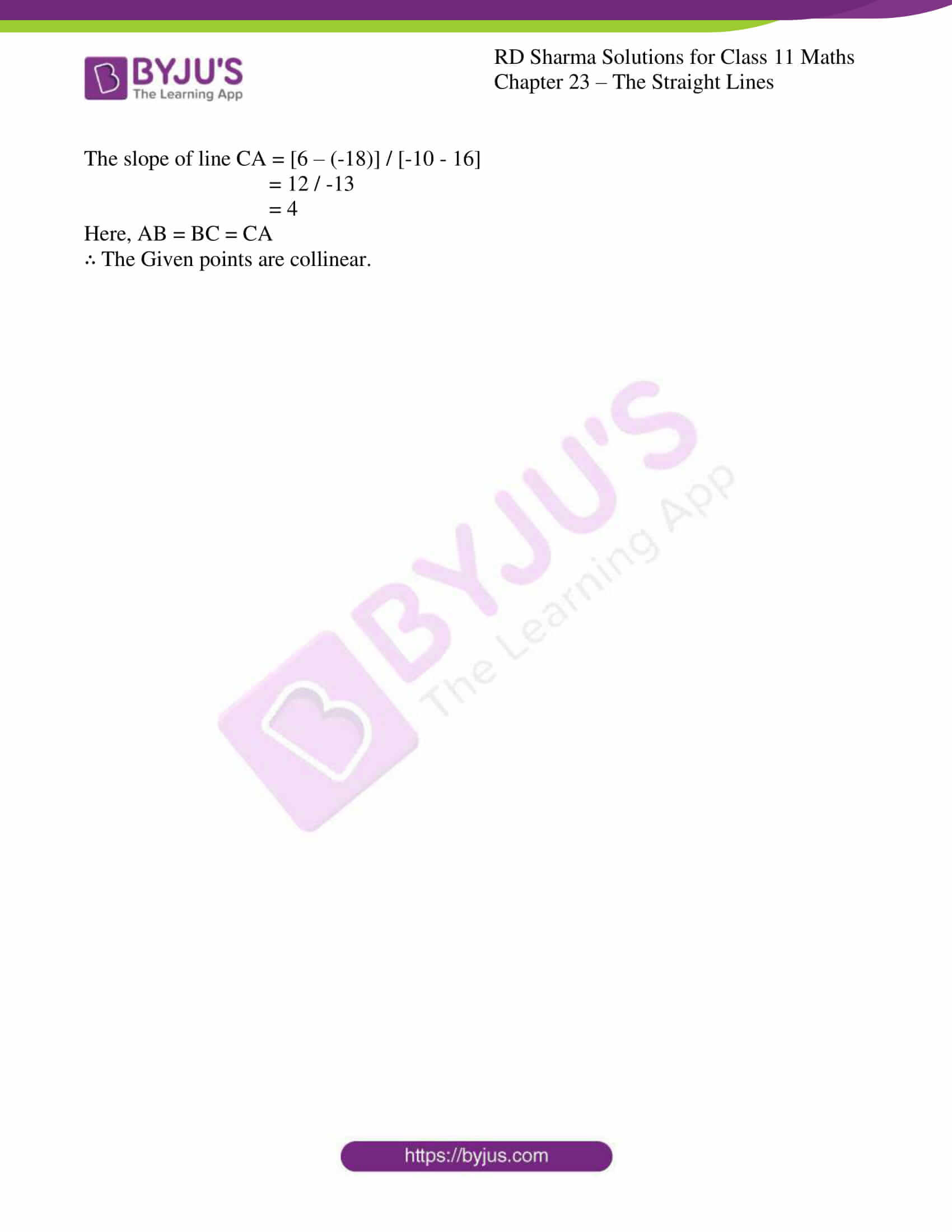### Access answers to RD Sharma Solutions for Class 11 Maths Exercise 23.1 Chapter 23 – The Straight Lines

#### EXERCISE 23.1 PAGE NO: 23.12

1. Find the slopes of the lines which make the following angles with the positive direction of x – axis:

(i) – π/4

(ii) 2π/3

Solution:

(i) – π/4

Let the slope of the line be ‘m’

Where, m = tan θ

So, the slope of Line is m = tan (– π/4)

= – 1

∴ The slope of the line is – 1.

(ii) 2π/3

Let the slope of the line be ‘m’

Where, m = tan θ

So, the slope of Line is m = tan (2π/3)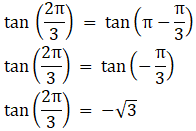∴ The slope of the line is –3

2. Find the slopes of a line passing through the following points :
(i) (–3, 2) and (1, 4)

(ii) (at21, 2at1) and (at22, 2at2)

Solution:

(i) (–3, 2) and (1, 4)

By using the formula,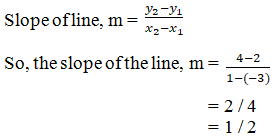∴ The slope of the line is ½.

(ii) (at21, 2at1) and (at22, 2at2)

By using the formula,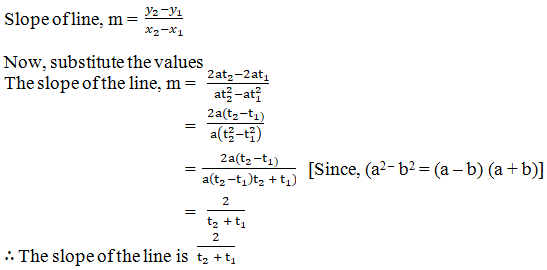3. State whether the two lines in each of the following are parallel, perpendicular or neither:
(i) Through (5, 6) and (2, 3); through (9, –2) and (6, –5)

(ii) Through (9, 5) and (– 1, 1); through (3, –5) and (8, –3)

Solution:

(i) Through (5, 6) and (2, 3); through (9, –2) and (6, –5)

By using the formula,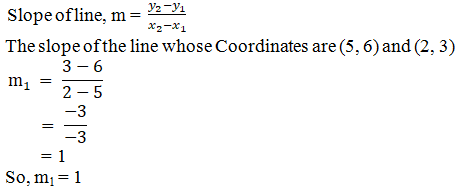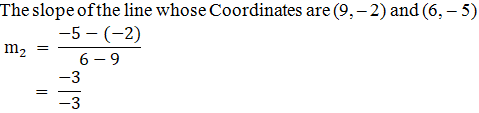So, m2 = 1

Here, m1 = m2 = 1

∴ The lines are parallel to each other.

(ii) Through (9, 5) and (– 1, 1); through (3, –5) and (8, –3)

By using the formula,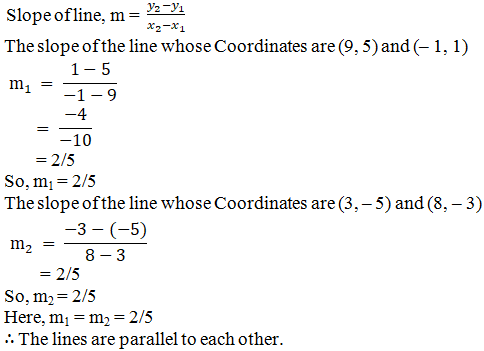4. Find the slopes of a line
(i) which bisects the first quadrant angle
(ii) which makes an angle of 300 with the positive direction of y – axis measured anticlockwise.

Solution:

(i) Which bisects the first quadrant angle?

Given: Line bisects the first quadrant

We know that, if the line bisects in the first quadrant, then the angle must be between line and the positive direction of x – axis.

Since, angle = 90/2 = 45o

By using the formula,

The slope of the line, m = tan θ

The slope of the line for a given angle is m = tan 45o

So, m = 1

∴ The slope of the line is 1.

(ii) Which makes an angle of 300 with the positive direction of y – axis measured anticlockwise?

Given: The line makes an angle of 30o with the positive direction of y – axis.

We know that, angle between line and positive side of axis => 90o + 30o = 120o

By using the formula,

The slope of the line, m = tan θ

The slope of the line for a given angle is m = tan 120o

So, m = – √3

∴ The slope of the line is – √3.

5. Using the method of slopes show that the following points are collinear:
(i) A (4, 8), B (5, 12), C (9, 28)

(ii) A(16, – 18), B(3, – 6), C(– 10, 6)

Solution:

(i) A (4, 8), B (5, 12), C (9, 28)

By using the formula,

The slope of the line = [y2 – y1] / [x2 – x1]

So,

The slope of line AB = [12 – 8] / [5 – 4]

= 4 / 1

The slope of line BC = [28 – 12] / [9 – 5]

= 16 / 4

= 4

The slope of line CA = [8 – 28] / [4 – 9]

= -20 / -5

= 4

Here, AB = BC = CA

∴ The Given points are collinear.

(ii) A(16, – 18), B(3, – 6), C(– 10, 6)

By using the formula,

The slope of the line = [y2 – y1] / [x2 – x1]

So,

The slope of line AB = [-6 – (-18)] / [3 – 16]

= 12 / -13

The slope of line BC = [6 – (-6)] / [-10 – 3]

= 12 / -13

The slope of line CA = [6 – (-18)] / [-10 – 16]

= 12 / -13

= 4

Here, AB = BC = CA

∴ The Given points are collinear.